## Nine-Point CircleThe Circle, also called Euler's Circle and the Feuerbach Circle, which passes through the feet of the Perpendicular,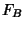, anddropped from the Vertices of any Triangleon the sides opposite them. Eulershowed in 1765 that it also passes through the Midpoints,,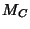of the sides of.

By Feuerbach's Theorem, the nine-point circle also passes through the Midpoints,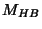,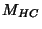of the segments which join the Vertices and the Orthocenter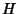. These three triples of points make nine in all, giving the circle its name. The centerof the nine-point circle is called the Nine-Point Center.

The Radius of the nine-point circle is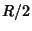, where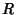is the Circumradius. The center of Kiepert's Hyperbola lies on the nine-point circle. The nine-point circle bisects any line from the Orthocenter to a point on the Circumcircle. The nine-point circle of the Incenter and Excenters of a Triangle is the Circumcircle.

The sum of the powers of the Vertices with regard to the nine-point circle isAlso,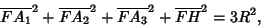whereis the Nine-Point Center,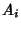are the Vertices,is the Orthocenter, andis the Circumradius. All triangles inscribed in a given Circle and having the same Orthocenter have the same nine-point circle.

See also Complete Quadrilateral, Eight-Point Circle Theorem, Feuerbach's Theorem, Fontené Theorems, Griffiths' Theorem, Nine-Point Center, Nine-Point Conic, Orthocentric System

References

Altshiller-Court, N. College Geometry: A Second Course in Plane Geometry for Colleges and Normal Schools, 2nd ed., rev. enl. New York: Barnes and Noble, pp. 93-97, 1952.

Brand, L. The Eight-Point Circle and the Nine-Point Circle.'' Amer. Math. Monthly 51, 84-85, 1944.

Coxeter, H. S. M. and Greitzer, S. L. Geometry Revisited. New York: Random House, pp. 20-22, 1967.

Dörrie, H. The Feuerbach Circle.'' §28 in 100 Great Problems of Elementary Mathematics: Their History and Solutions. New York: Dover, pp. 142-144, 1965.

Gardner, M. Mathematical Carnival: A New Round-Up of Tantalizers and Puzzles from Scientific American. New York: Vintage Books, p. 59, 1977.

Guggenbuhl, L. Karl Wilhelm Feuerbach, Mathematician.'' Appendix to Circles: A Mathematical View, rev. ed. Washington, DC: Math. Assoc. Amer., pp. 89-100, 1995.

Johnson, R. A. Modern Geometry: An Elementary Treatise on the Geometry of the Triangle and the Circle. Boston, MA: Houghton Mifflin, pp. 165 and 195-212, 1929.

Lange, J. Geschichte des Feuerbach'schen Kreises. Berlin, 1894.

Mackay, J. S. History of the Nine-Point Circle.'' Proc. Edinburgh Math. Soc. 11, 19-61, 1892.

Ogilvy, C. S. Excursions in Geometry. New York: Dover, pp. 119-120, 1990.

Pedoe, D. Circles: A Mathematical View, rev. ed. Washington, DC: Math. Assoc. Amer., pp. 1-4, 1995.